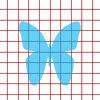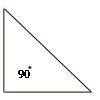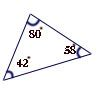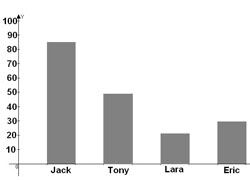## Sep 8, 2009

This free math worksheet contains practice questions for grade 4 students. Keystage 1 students can also practice this question paper.

1.   Find the place value of 9 in 348.719.
a.   9 hundredths
b.   9 thousandths
c.   9 hundreds
d.   9 thousands
2.   Which is the greatest 2 digit prime number?
a.   99
b.   97
c.   91
d.   83
3.   30 cents+2 dimes=________
a.   2 quarters
b.   4 nickels
c.   10 dimes
d.   100 cents
4.   The Math test scores of Grade 4 students are given below:
90, 56, 36, 55, 99, 55, 67, 42, 51, 63 and 65.
Find the range of the above data.
a.   99
b.   36
c.   63
d.   55
5.   A shelf contains 7 boxes. There are 12 pencils in a box. How many pencils are there in total?
a.   19
b.   5
c.   84
d.   14
6.   Estimate the area of a picture in the given grid:a.   18 sq. units
b.   14 sq. units
c.   12 sq. units
d.   8 sq. units
7.   5 ft 3 in + 2 ft 5 in is equal to ______
a.   7 ft 8 in
b.   8 ft 7 in
c.   3 ft 2 in
d.   0 ft 2 in
8.   2 cm=___ mm
a.   10
b.   20
c.   100
d.   200
9.   There are 8000 grams of rice in a container. Dawn wanted to make 1 kg of rice in each bag. How many bags are required to empty the container?
a.   8000
b.   800
c.   80
d.   8
10.The given triangle is _________
a.   Equilateral triangle
b.   Isosceles triangle
c.   Right triangle
d.   Scalene triangle
11.  In a rectangle, which of the following statement is not true?
a.   Sides are equal
b.   The angles are 90 degree
c.   Diagonals bisect each other
d.   Diagonals are equal
12. If a ray makes an angle 50 degree with a straight line, the angle formed is _____
a.   Acute angle
b.   Obtuse angle
c.   Right angle
d.   Straight angle
13.The given triangle is ________triangle
a.   Acute
b.   Obtuse
c.   Right
d.   Straight
14. Fill in the missing number in the given number sequence:
1, 4, 10, 13, 16, ___, 22, 25.
a.   17
b.   18
c.   19
d.   20
15. Choose the correct expression for the given statement:
Cathy has n number of apples. He gave away 3 of the apples to his friend.
a.   n+3
b.   3n
c.   n-3
d.   3
16. Jason filled 6 cups of water in the first can. 12 cups of water in the second can and 18 cups of water in the third can. If the pattern continues, which can is filled with 36 cups of water?
a.     4
b.   5
c.   6
d.   7
17.  If y=3+x and x takes the value as 4, then the value of y is ______
a.   7
b.   6
c.   5
d.   4
18.There are 5 green color beads and 6 yellow color beads in a box. Find the probability of selecting a green bead from the box.
a.   5/6
b.   1/11
c.   6/5
d.   1/5
19. Find the median for the given data:
45, 22, 76, 90, 35, 78,92
a.   45
b.   76
c.   78
d.   90
20. The bar graph given below shows the Math scores of grade 4 classmates. Who is in the second highest position?a.   Jack
b.   Tony
c.   Lara
d.   Eric
Previous post:
Next post:
Related articles: